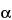Search USP29

GLOSSARY OF SYMBOLS
To promote uniformity of interpretation, the following symbols and definitions are employed where applicable in presenting formulas in the individual monographs. [NOTE—Where the terms W and t both appear in the same equation they must be expressed in the same units.]relative retention, cR, cI, cU concentrations of Reference Standard, internal standard, and analyte in a particular solution. CA concentration ratio of analyte and internal standard in test solution or Assay preparation, CS concentration ratio of Reference Standard and internal standard in Standard solution, f distance from the peak maximum to the leading edge of the peak, the distance being measured at a point 5% of the peak height from the baseline. k¢ capacity factor, N number of theoretical plates in a chromatographic column, qR, qI, qU total quantities (weights) of ReferenceStandard, internal standard, and analyte ina particular solution. QA quantity ratio of analyte and internal standard in test solution or Assay preparation, QS quantity ratio of Reference Standard and internal standard in Standard solution, rS peak response of the Reference Standard obtained from a chromatogram. rU peak response of the analyte obtained from a chromatogram. R resolution between two chromatographic peaks, RF chromatographic retardation factor equal to the ratio of the distance from the origin to the center of a zone divided by the distance from the origin to the solvent front. RR relative retention RR relative retention time RS peak response ratio for Standard preparation containing Reference Standard and internal standard, RU peak response ratio for Assay preparation containing the analyte and internal standard, SR (%) relative standard deviation in percentage, where Xi is an individual measurement in a set of N measurements and X is the arithmetic mean of the set. T tailing factor, t retention time measured from time of injection to time of elution of peak maximum. ta retention time of nonretarded component, air with thermal conductivity detection. W width of peak measured by extrapolating the relatively straight sides to the baseline. Wh/2 width of peak at half height. W0.05 width of peak at 5% height.